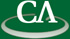## Polynomials from a Computational Perspective

### Manindra Agrawal, Department of Computer Science and Engineering, IIT Kanpur

Thursday June 13th, 10:30am, IRMACS theatre, ASB Room 10900.

```Polynomials are one of the fundamental objects in mathematics. In this
talk, we focus on the problem of classifying polynomials. We argue for
classifying polynomials according to the complexity of computing them.
This notion is formalized as arithmetic complexity of a polynomial or
polynomial family. After discussing some examples, we identify two
important classes of polynomials according to their arithmetic
complexity: VP and VNP. These are analogs of the classes P and NP in
the algebraic settings, and it is not known if VP equals VNP. We
connect this question with the problem of deciding if two arithmetic
circuits compute the same polynomial, and review the exciting recent
progress made on solving this problem.

```